Скачать презентацию On the Hardness of Inferring Phylogenies from Triplet-Dissimilarities

e2fe7787c004b2db2c1ceb19994359b3.ppt

• Количество слайдов: 30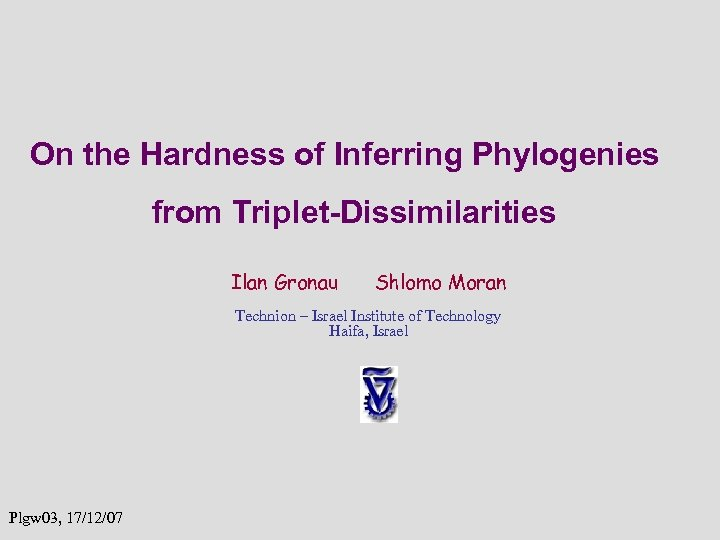On the Hardness of Inferring Phylogenies from Triplet-Dissimilarities Ilan Gronau Shlomo Moran Technion – Israel Institute of Technology Haifa, Israel Plgw 03, 17/12/07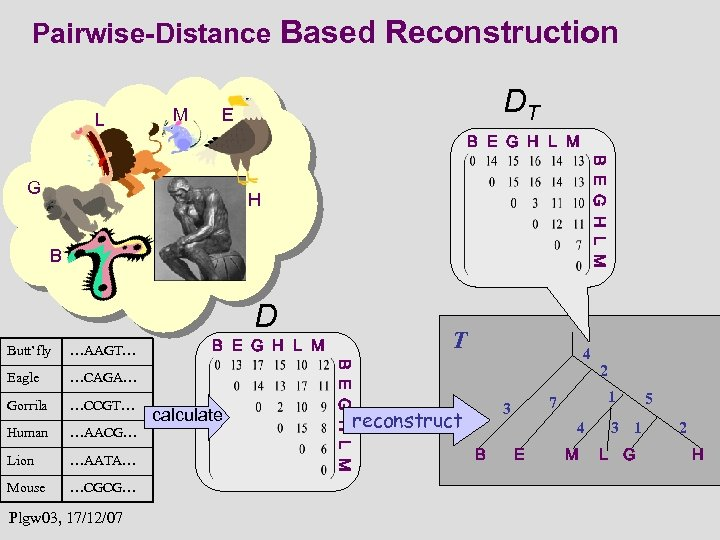Pairwise-Distance Based Reconstruction L M DT E B E G H L M G H B D …AAGT… Eagle …CAGA… Gorrila …CCGT… Human …AACG… Lion …AATA… Mouse …CGCG… Plgw 03, 17/12/07 T B E G H L M calculate B E G H L M Butt’fly 4 reconstruct 1 7 3 4 B E 2 M 3 1 L G 5 2 H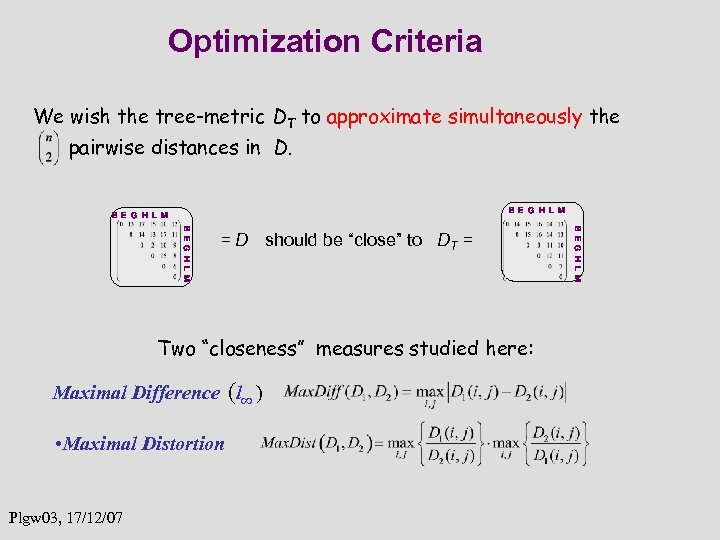Optimization Criteria We wish the tree-metric DT to approximate simultaneously the pairwise distances in D. B E G H L M Two “closeness” measures studied here: Maximal Difference (l∞ ) • Maximal Distortion Plgw 03, 17/12/07 B E G H L M = D should be “close” to DT =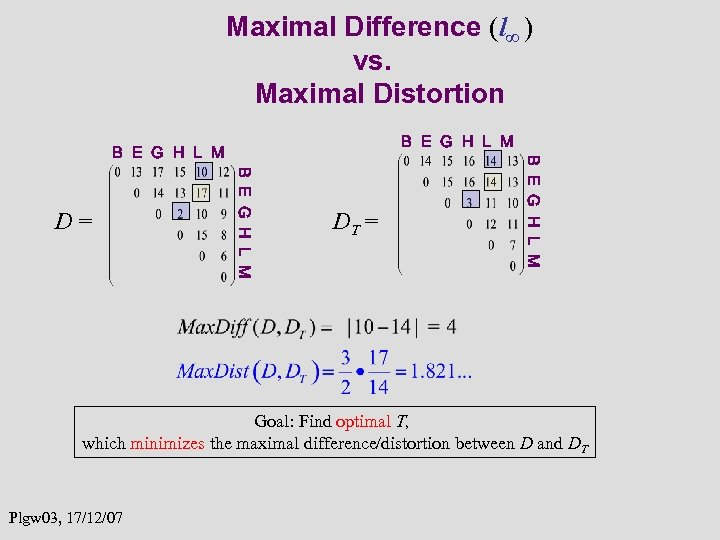Maximal Difference (l∞ ) vs. Maximal Distortion B E G H L M D= DT = B E G H L M Goal: Find optimal T, which minimizes the maximal difference/distortion between D and DT Plgw 03, 17/12/07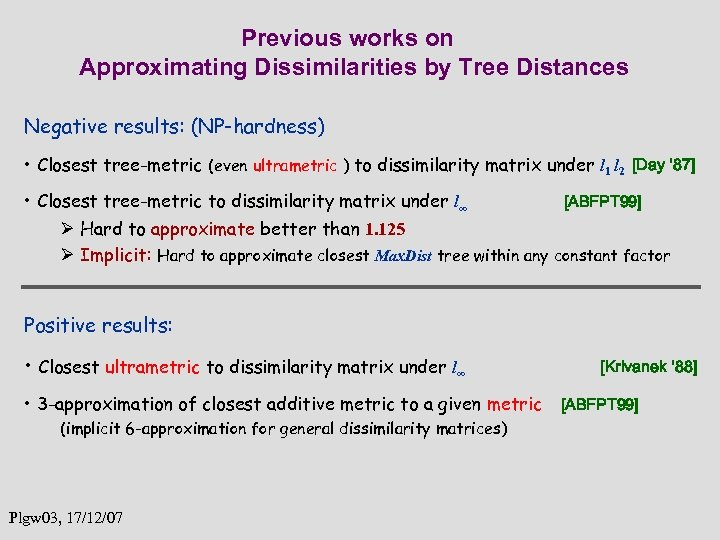Previous works on Approximating Dissimilarities by Tree Distances Negative results: (NP-hardness) • Closest tree-metric (even ultrametric ) to dissimilarity matrix under l 1 l 2 [Day ‘ 87] • Closest tree-metric to dissimilarity matrix under l∞ [ABFPT 99] Ø Hard to approximate better than 1. 125 Ø Implicit: Hard to approximate closest Max. Dist tree within any constant factor Positive results: • Closest ultrametric to dissimilarity matrix under l∞ • 3 -approximation of closest additive metric to a given metric (implicit 6 -approximation for general dissimilarity matrices) Plgw 03, 17/12/07 [Krivanek ‘ 88] [ABFPT 99]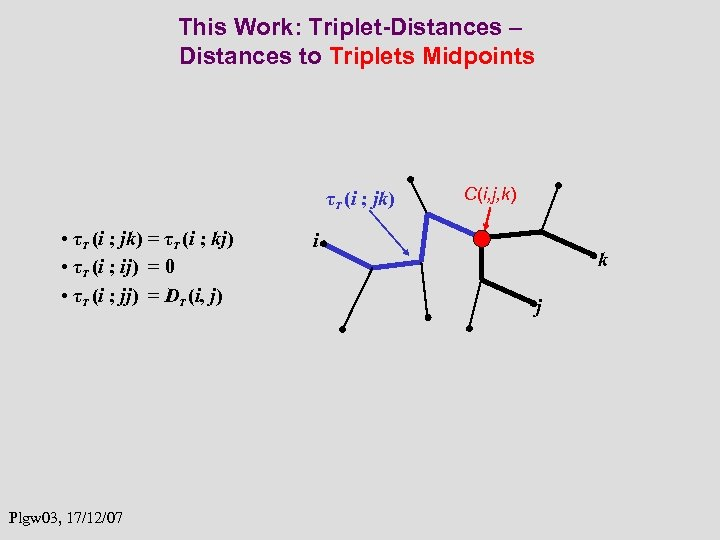This Work: Triplet-Distances – Distances to Triplets Midpoints τT (i ; jk) • τT (i ; jk) = τT (i ; kj) • τT (i ; ij) = 0 • τT (i ; jj) = DT (i, j) Plgw 03, 17/12/07 C(i, j, k) i k j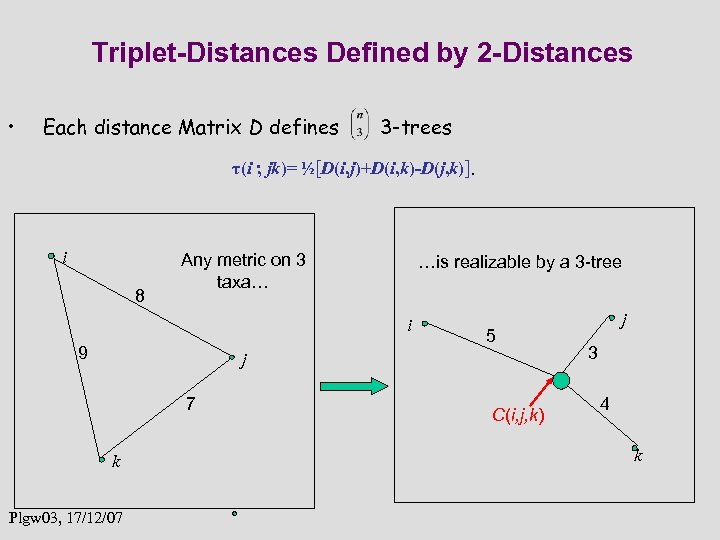Triplet-Distances Defined by 2 -Distances • Each distance Matrix D defines 3 -trees τ(i ; jk)= ½[D(i, j)+D(i, k)-D(j, k)]. i 8 Any metric on 3 taxa… …is realizable by a 3 -tree i 9 5 j 7 k Plgw 03, 17/12/07 C(i, j, k) j 3 4 k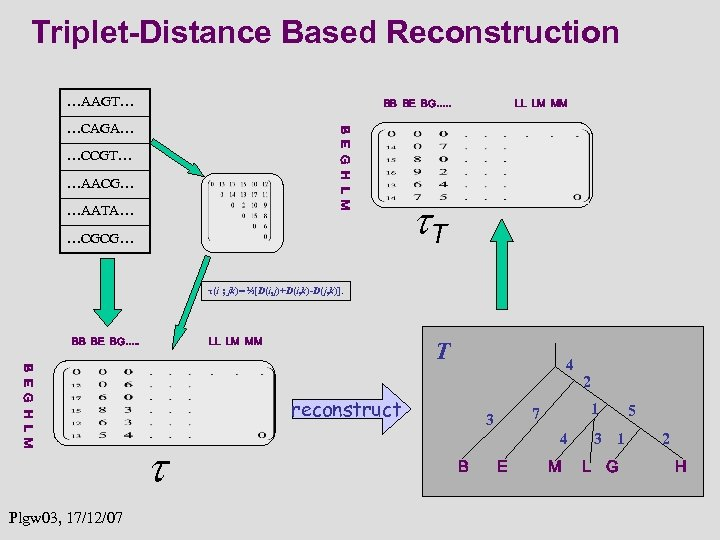Triplet-Distance Based Reconstruction …AAGT… BB BE BG…. . B E G H L M …CAGA… …CCGT… …AACG… …AATA… …CGCG… LL LM MM T τ(i ; jk)= ½[D(i, j)+D(i, k)-D(j, k)]. BB BE BG…. . B E G H L M Plgw 03, 17/12/07 LL LM MM T 4 reconstruct 1 7 3 4 B E 2 M 3 1 L G 5 2 H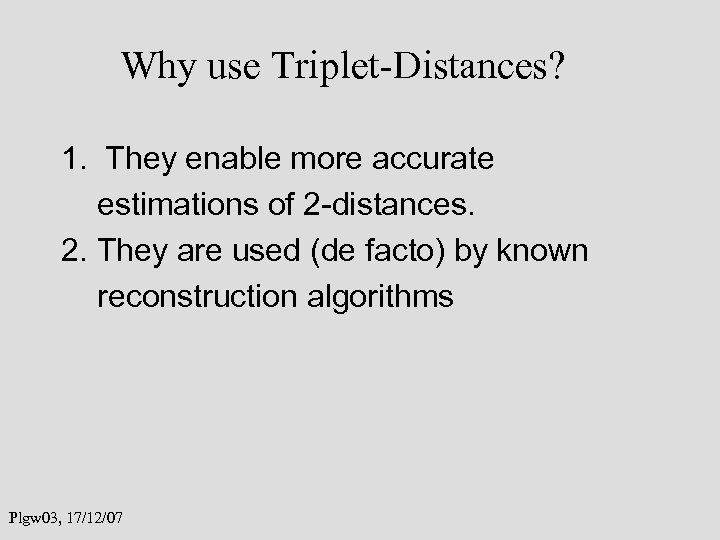Why use Triplet-Distances? 1. They enable more accurate estimations of 2 -distances. 2. They are used (de facto) by known reconstruction algorithms Plgw 03, 17/12/07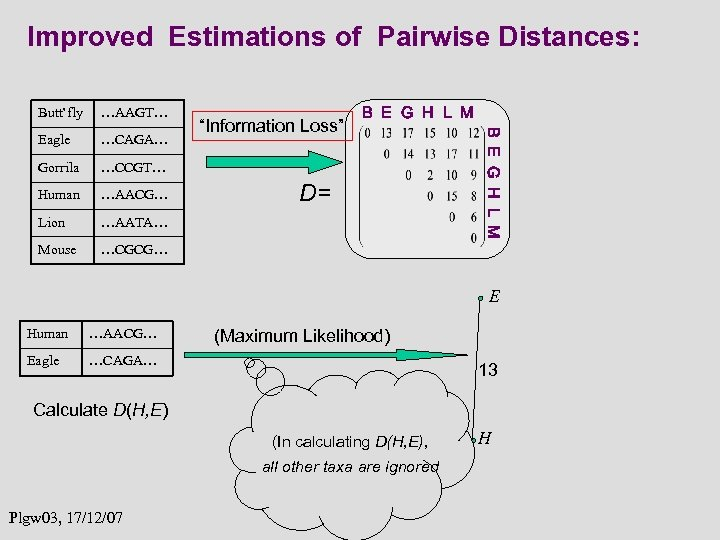Improved Estimations of Pairwise Distances: …AAGT… Eagle …CAGA… Gorrila …CCGT… Human …AACG… Lion …AATA… Mouse B E G H L M …CGCG… “Information Loss” D= B E G H L M Butt’fly E Human …AACG… Eagle …CAGA… (Maximum Likelihood) 13 Calculate D(H, E) (In calculating D(H, E), all other taxa are ignored Plgw 03, 17/12/07 H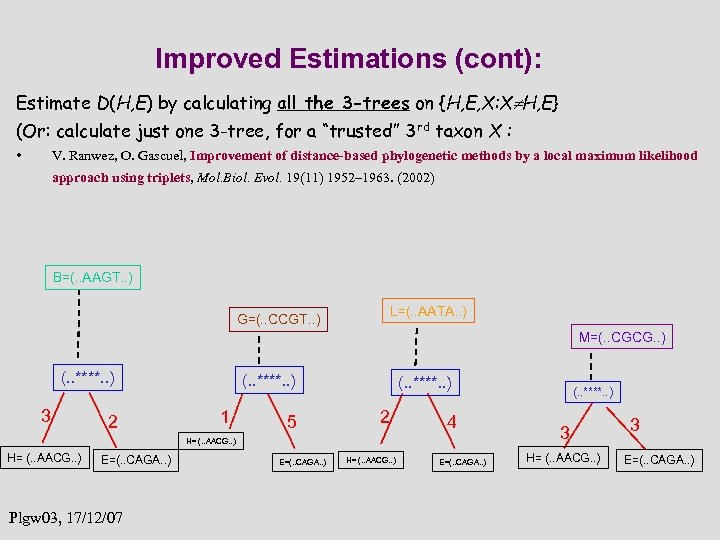Improved Estimations (cont): Estimate D(H, E) by calculating all the 3 -trees on {H, E, X: X H, E} (Or: calculate just one 3 -tree, for a “trusted” 3 rd taxon X : • V. Ranwez, O. Gascuel, Improvement of distance-based phylogenetic methods by a local maximum likelihood approach using triplets, Mol. Biol. Evol. 19(11) 1952– 1963. (2002) B=(. . AAGT. . ) G=(. . CCGT. . ) L=(. . AATA. . ) M=(. . CGCG. . ) (. . ****. . ) 3 2 (. . ****. . ) 1 5 (. . ****. . ) 2 4 H= (. . AACG. . ) E=(. . CAGA. . ) Plgw 03, 17/12/07 E=(. . CAGA. . ) H= (. . AACG. . ) E=(. . CAGA. . ) (. . ****. . ) 3 H= (. . AACG. . ) 3 E=(. . CAGA. . )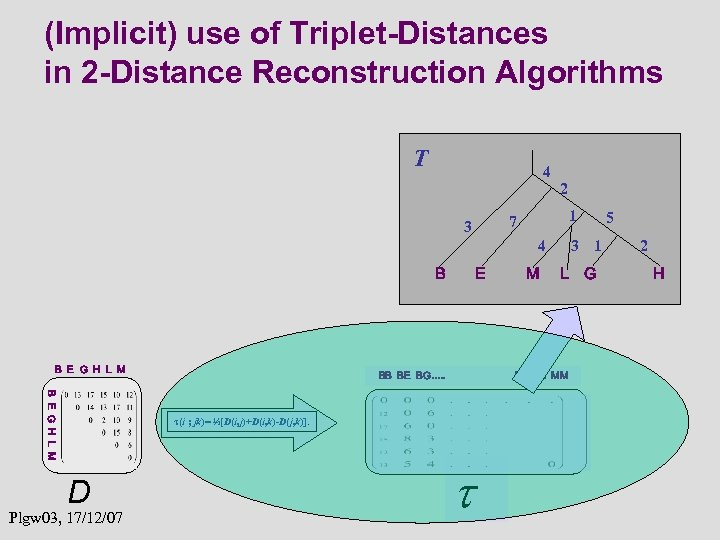(Implicit) use of Triplet-Distances in 2 -Distance Reconstruction Algorithms T 4 2 1 7 3 4 B B E G H L M E BB BE BG…. . B E G H L M Plgw 03, 17/12/07 L G LL LM MM τ(i ; jk)= ½[D(i, j)+D(i, k)-D(j, k)]. D M 3 1 5 2 H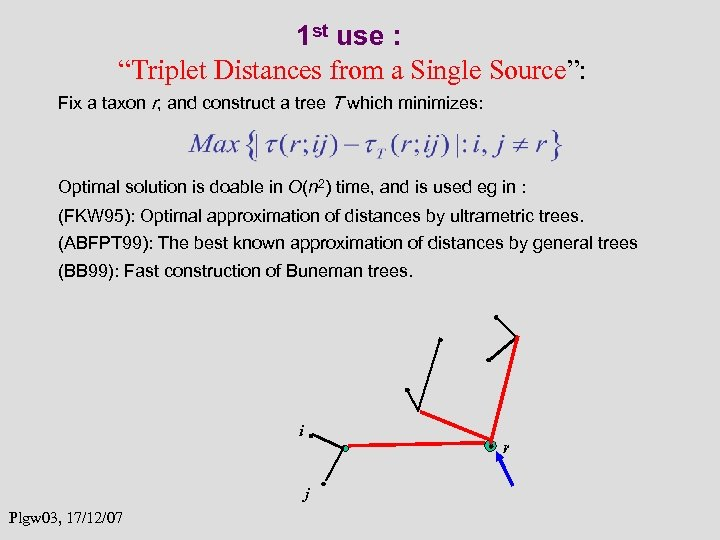1 st use : “Triplet Distances from a Single Source”: Fix a taxon r, and construct a tree T which minimizes: Optimal solution is doable in O(n 2) time, and is used eg in : (FKW 95): Optimal approximation of distances by ultrametric trees. (ABFPT 99): The best known approximation of distances by general trees (BB 99): Fast construction of Buneman trees. i r j Plgw 03, 17/12/07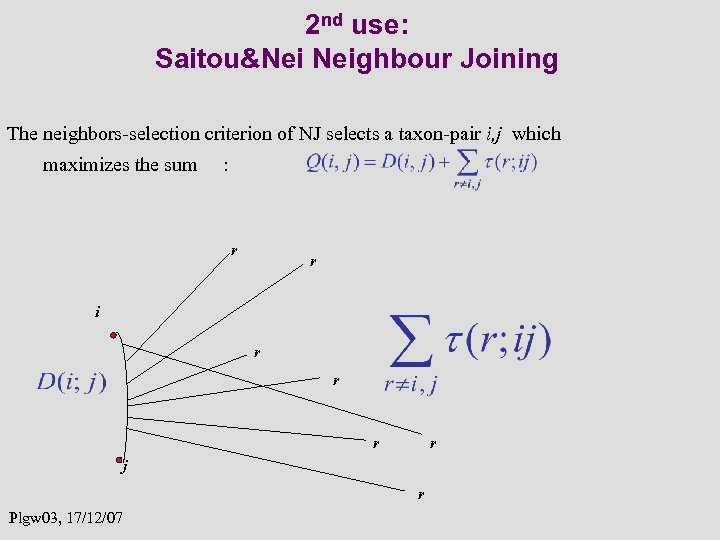2 nd use: Saitou&Nei Neighbour Joining The neighbors-selection criterion of NJ selects a taxon-pair i, j which maximizes the sum : r r i r r j r Plgw 03, 17/12/07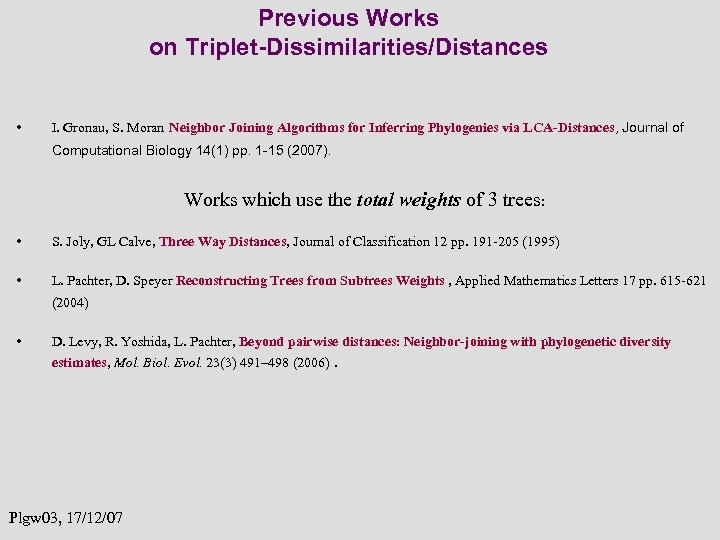Previous Works on Triplet-Dissimilarities/Distances • I. Gronau, S. Moran Neighbor Joining Algorithms for Inferring Phylogenies via LCA-Distances, Journal of Computational Biology 14(1) pp. 1 -15 (2007). Works which use the total weights of 3 trees: • S. Joly, GL Calve, Three Way Distances, Journal of Classification 12 pp. 191 -205 (1995) • L. Pachter, D. Speyer Reconstructing Trees from Subtrees Weights , Applied Mathematics Letters 17 pp. 615 -621 (2004) • D. Levy, R. Yoshida, L. Pachter, Beyond pairwise distances: Neighbor-joining with phylogenetic diversity estimates, Mol. Biol. Evol. 23(3) 491– 498 (2006). Plgw 03, 17/12/07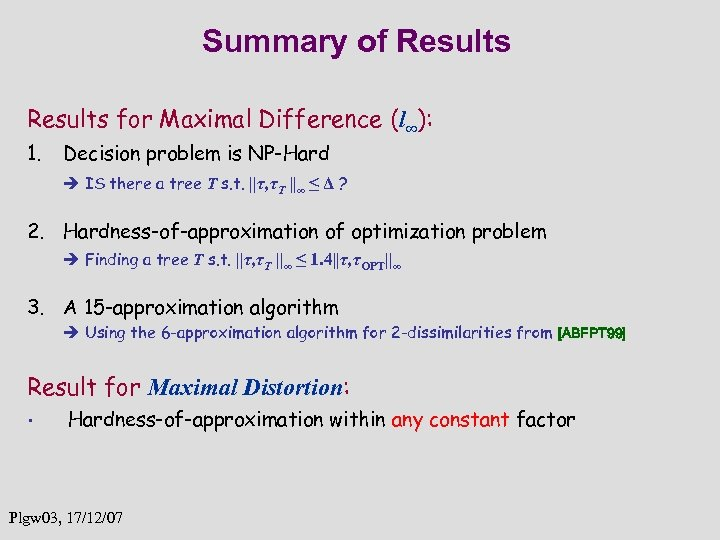Summary of Results for Maximal Difference (l∞): 1. Decision problem is NP-Hard IS there a tree T s. t. ||τ, τT ||∞ ≤ Δ ? 2. Hardness-of-approximation of optimization problem Finding a tree T s. t. ||τ, τT ||∞ ≤ 1. 4||τ, τOPT||∞ 3. A 15 -approximation algorithm Using the 6 -approximation algorithm for 2 -dissimilarities from [ABFPT 99] Result for Maximal Distortion: • Hardness-of-approximation within any constant factor Plgw 03, 17/12/07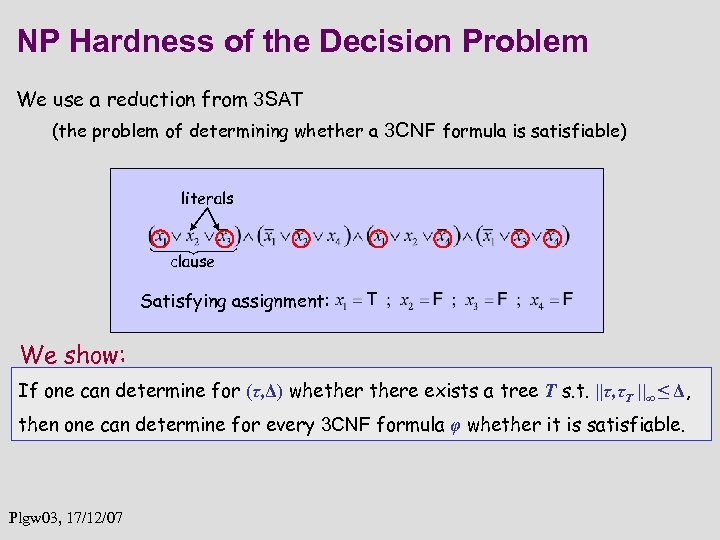NP Hardness of the Decision Problem We use a reduction from 3 SAT (the problem of determining whether a 3 CNF formula is satisfiable) literals clause Satisfying assignment: We show: If one can determine for (τ, Δ) whethere exists a tree T s. t. ||τ, τT ||∞ ≤ Δ, then one can determine for every 3 CNF formula φ whether it is satisfiable. Plgw 03, 17/12/07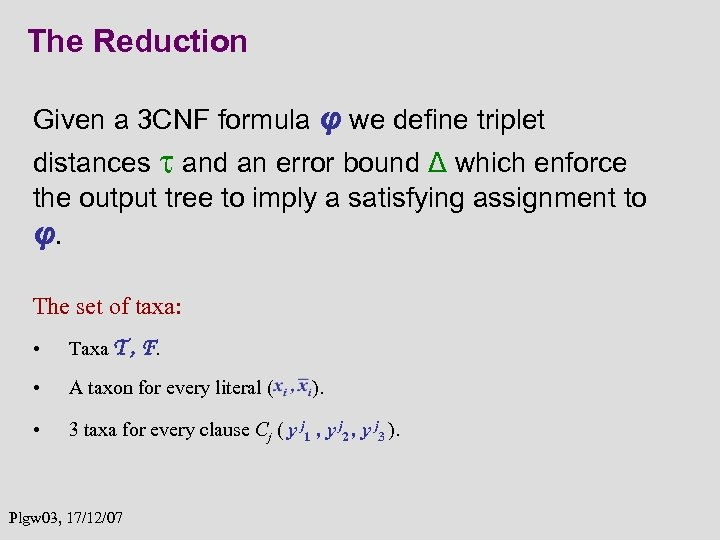The Reduction Given a 3 CNF formula φ we define triplet distances and an error bound Δ which enforce the output tree to imply a satisfying assignment to φ. The set of taxa: • Taxa T , F. • A taxon for every literal ( • 3 taxa for every clause Cj ( y j 1 , y j 2 , y j 3 ). Plgw 03, 17/12/07 ).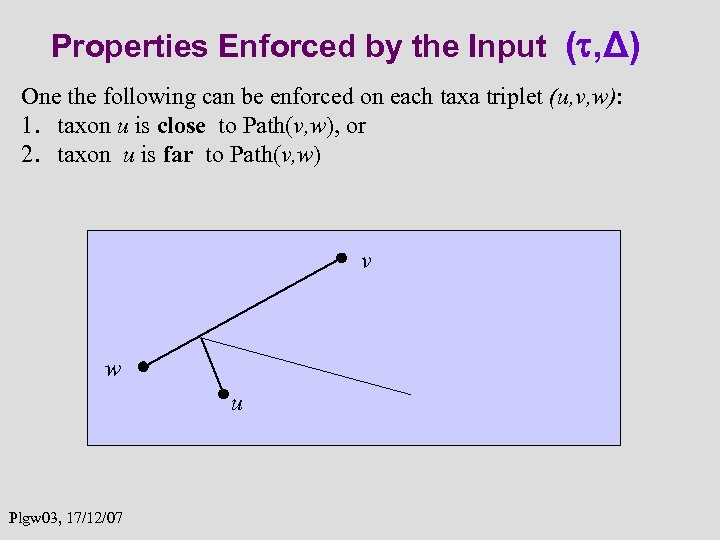Properties Enforced by the Input ( , Δ) One the following can be enforced on each taxa triplet (u, v, w): 1. taxon u is close to Path(v, w), or 2. taxon u is far to Path(v, w) v w u Plgw 03, 17/12/07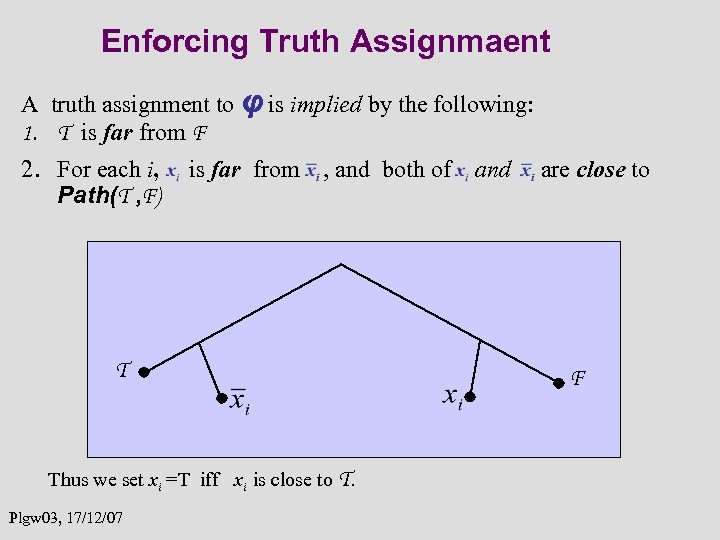Enforcing Truth Assignmaent A truth assignment to φ is implied by the following: 1. T is far from F 2. For each i, Path(T , F) is far from , and both of T Thus we set xi =T iff xi is close to T. Plgw 03, 17/12/07 and are close to F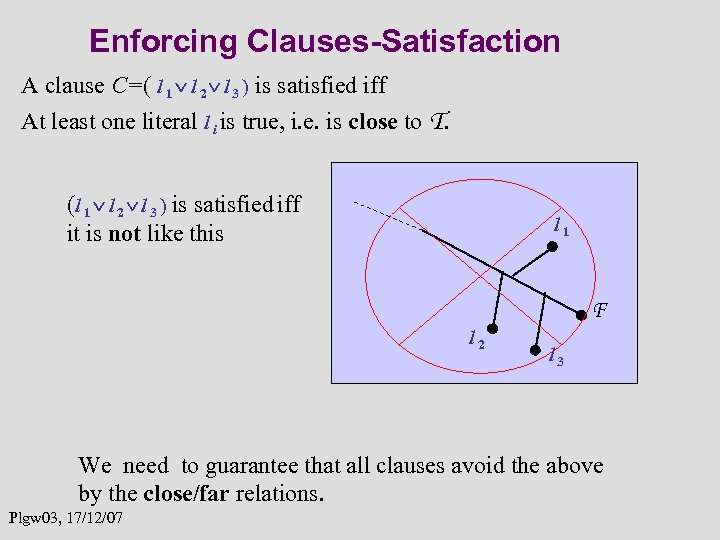Enforcing Clauses-Satisfaction A clause C=( l 1 l 2 l 3 ) is satisfied iff At least one literal l i is true, i. e. is close to T. (l 1 l 2 l 3 ) is satisfied iff it is not like this l 1 F l 2 l 3 We need to guarantee that all clauses avoid the above by the close/far relations. Plgw 03, 17/12/07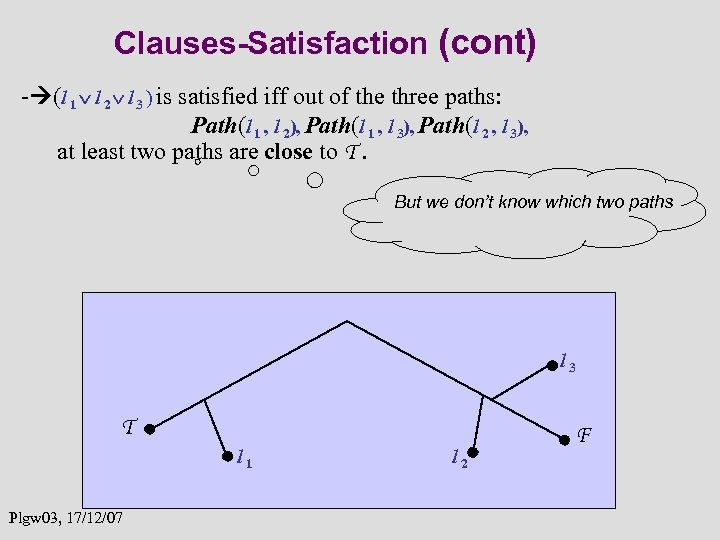Clauses-Satisfaction (cont) - (l 1 l 2 l 3 ) is satisfied iff out of the three paths: Path(l 1 , l 2), Path(l 1 , l 3), Path(l 2 , l 3), at least two paths are close to T. But we don’t know which two paths l 3 T l 1 Plgw 03, 17/12/07 l 2 F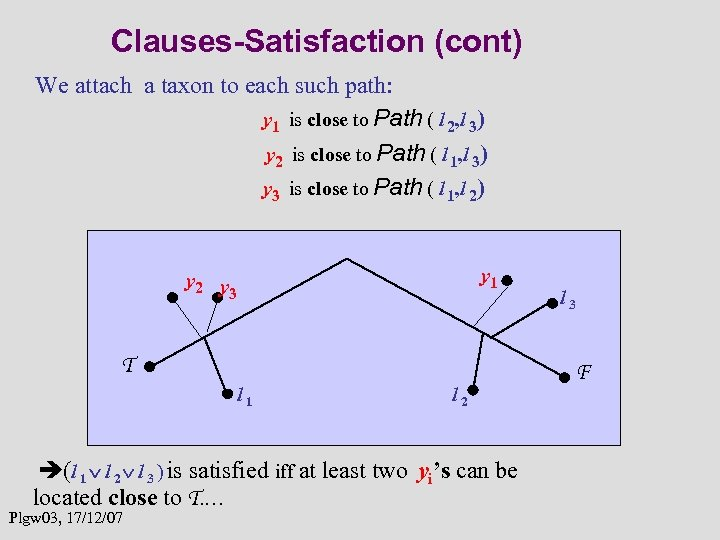Clauses-Satisfaction (cont) We attach a taxon to each such path: y 1 is close to Path ( l 2, l 3) y 2 is close to Path ( l 1, l 3) y 3 is close to Path ( l 1, l 2) y 1 y 2 y 3 T l 1 l 2 (l 1 l 2 l 3 ) is satisfied iff at least two yi’s can be located close to T. … Plgw 03, 17/12/07 l 3 F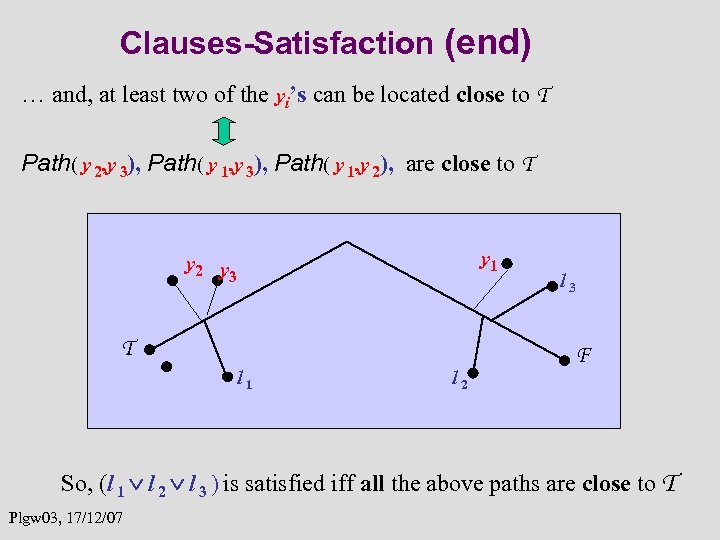Clauses-Satisfaction (end) … and, at least two of the yi’s can be located close to T Path( y 2, y 3), Path( y 1, y 2), are close to T y 1 y 2 y 3 T l 1 l 2 l 3 F So, (l 1 l 2 l 3 ) is satisfied iff all the above paths are close to T Plgw 03, 17/12/07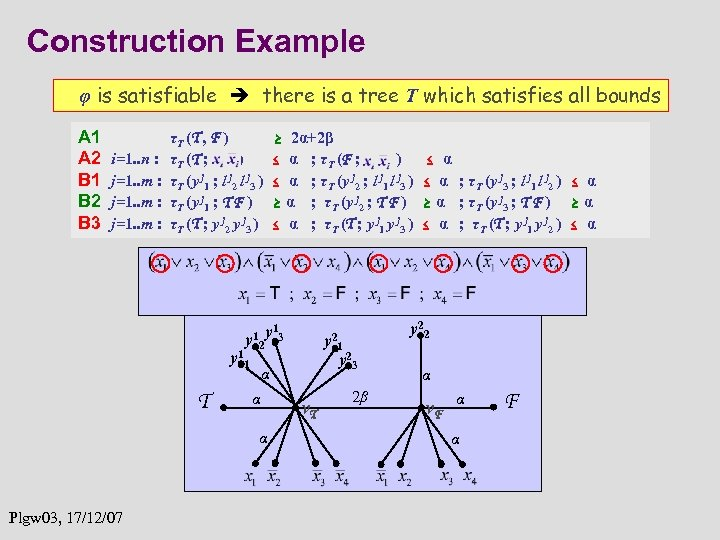Construction Example φ is satisfiable there is a tree T which satisfies all bounds A 1 A 2 B 1 B 2 B 3 i=1. . n : j=1. . m : ≥ 2α+2β ≤ α ; τT (F ; ) ≤ α ; τT (y j 2 ; l j 1 l j 3 ) ≥ α ; τT (y j 2 ; T F ) ≤ α ; τT (T ; y j 1 y j 3 ) τT (T , F ) τT (T ; ) τT (y j 1 ; l j 2 l j 3 ) τT (y j 1 ; T F ) τT (T ; y j 2 y j 3 ) y 11 T 2 y 13 α y 22 1 y 23 α α Plgw 03, 17/12/07 y 2 v. T ≤ α ; τT (y j 3 ; l j 1 l j 2 ) ≤ α ≥ α ; τT (y j 3 ; T F ) ≥ α ≤ α ; τT (T ; y j 1 y j 2 ) ≤ α 2β α v. F α α F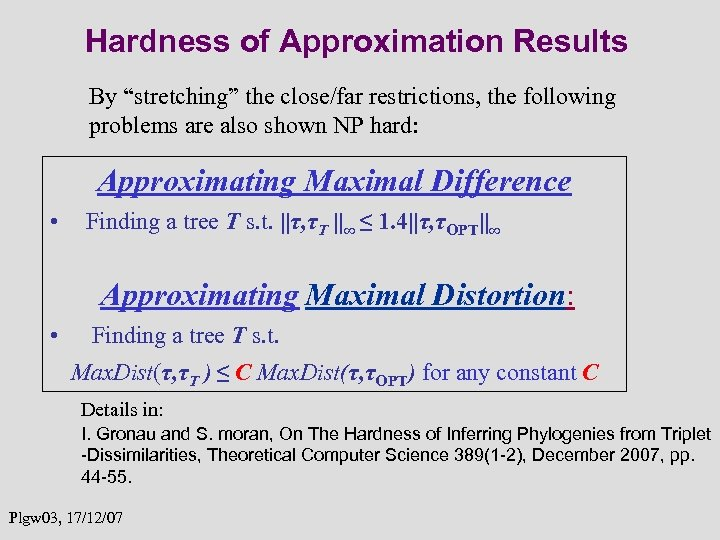Hardness of Approximation Results By “stretching” the close/far restrictions, the following problems are also shown NP hard: Approximating Maximal Difference • Finding a tree T s. t. ||τ, τT ||∞ ≤ 1. 4||τ, τOPT||∞ Approximating Maximal Distortion: • Finding a tree T s. t. Max. Dist(τ, τT ) ≤ C Max. Dist(τ, τOPT) for any constant C Details in: I. Gronau and S. moran, On The Hardness of Inferring Phylogenies from Triplet -Dissimilarities, Theoretical Computer Science 389(1 -2), December 2007, pp. 44 -55. Plgw 03, 17/12/07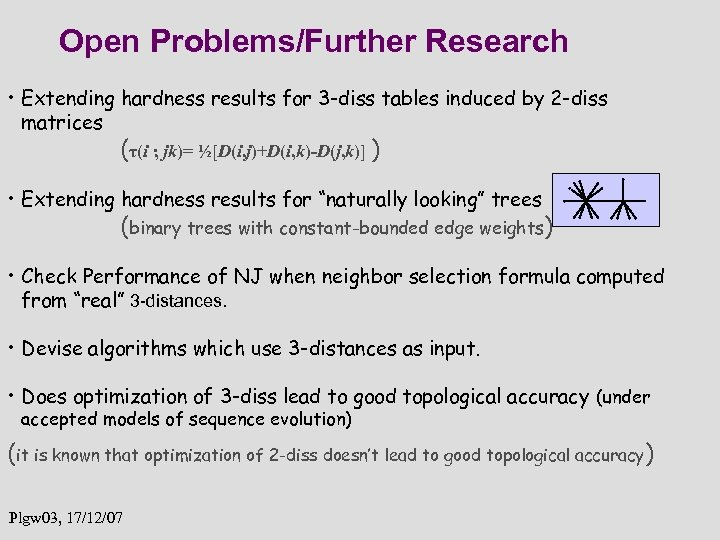Open Problems/Further Research • Extending hardness results for 3 -diss tables induced by 2 -diss matrices (τ(i ; jk)= ½[D(i, j)+D(i, k)-D(j, k)] ) • Extending hardness results for “naturally looking” trees (binary trees with constant-bounded edge weights) • Check Performance of NJ when neighbor selection formula computed from “real” 3 -distances. • Devise algorithms which use 3 -distances as input. • Does optimization of 3 -diss lead to good topological accuracy (under accepted models of sequence evolution) (it is known that optimization of 2 -diss doesn’t lead to good topological accuracy ) Plgw 03, 17/12/07Thank You Plgw 03, 17/12/07 28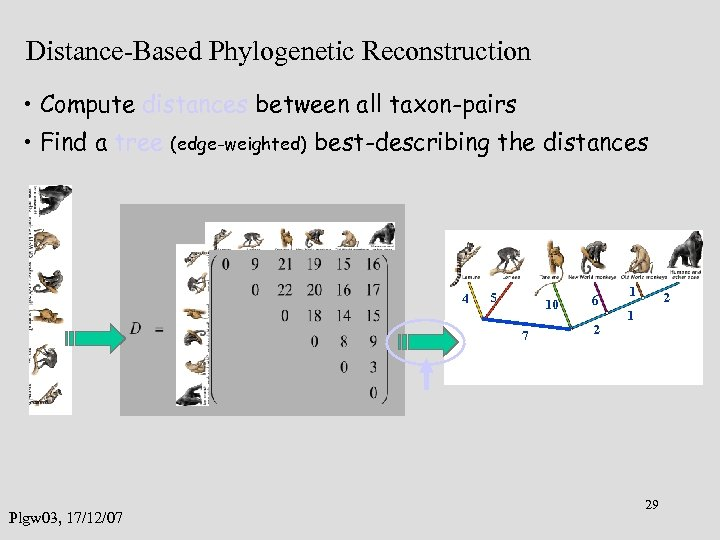Distance-Based Phylogenetic Reconstruction • Compute distances between all taxon-pairs • Find a tree (edge-weighted) best-describing the distances 4 5 10 7 Plgw 03, 17/12/07 6 2 1 29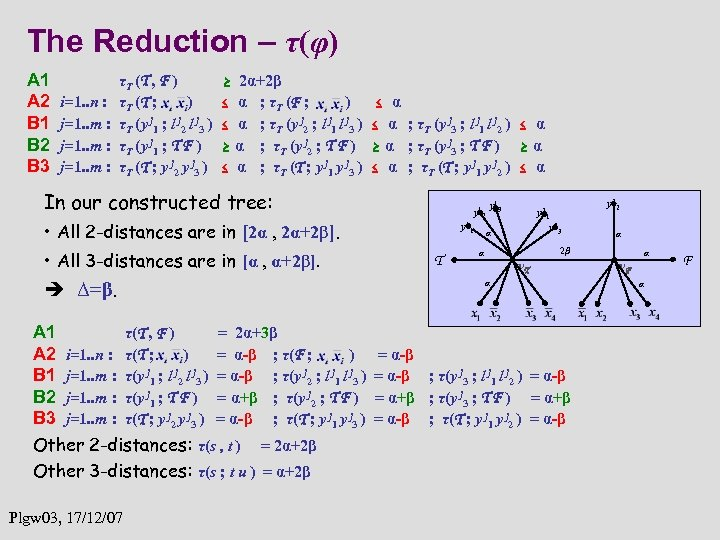The Reduction – τ(φ) A 1 A 2 B 1 B 2 B 3 i=1. . n : j=1. . m : τT (T , F ) τT (T ; ) τT (y j 1 ; l j 2 l j 3 ) τT (y j 1 ; T F ) τT (T ; y j 2 y j 3 ) ≥ 2α+2β ≤ α ; τT (F ; ) ≤ α ; τT (y j 2 ; l j 1 l j 3 ) ≥ α ; τT (y j 2 ; T F ) ≤ α ; τT (T ; y j 1 y j 3 ) ≤ α ; τT (y j 3 ; l j 1 l j 2 ) ≤ α ≥ α ; τT (y j 3 ; T F ) ≥ α ≤ α ; τT (T ; y j 1 y j 2 ) ≤ α In our constructed tree: • All 2 -distances are in [2α , 2α+2β]. • All 3 -distances are in [α , α+2β]. Δ=β. A 1 τ(T , F ) = 2α+3β A 2 i=1. . n : τ(T ; ) = α-β ; τ(F ; ) B 1 j=1. . m : τ(y j 1 ; l j 2 l j 3 ) = α-β ; τ(y j 2 ; l j 1 l j 3 ) B 2 j=1. . m : τ(y j 1 ; T F ) = α+β ; τ(y j 2 ; T F ) B 3 j=1. . m : τ(T ; y j 2 y j 3 ) = α-β ; τ(T ; y j 1 y j 3 ) Other 2 -distances: τ(s , t ) = 2α+2β Other 3 -distances: τ(s ; t u ) = α+2β Plgw 03, 17/12/07 y 12 y 11 T y 13 y 22 y 21 y 23 α α 2β α v. T α = α-β ; τ(y j 3 ; l j 1 l j 2 ) = α-β = α+β ; τ(y j 3 ; T F ) = α+β = α-β ; τ(T ; y j 1 y j 2 ) = α-β v. F α α F